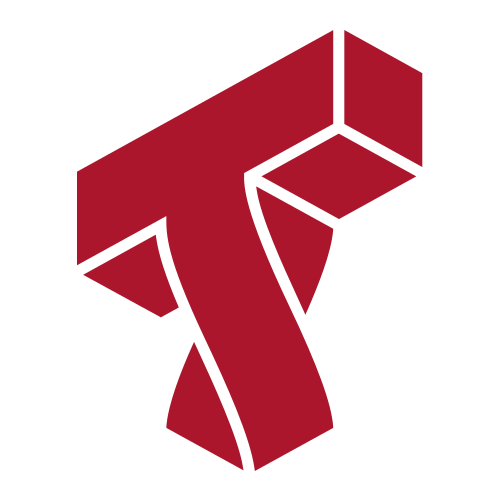# Lecture 23: Course Overview¶

### Applied Machine Learning¶

Volodymyr Kuleshov
Cornell Tech

# Congratulations on Finishing Applied Machine Learning!¶

You have made it! This is our last machine learning lecture, in which we will do an overview of the diffrent algorithms seen in the course.

# A Map of Applied Machine Learning¶

We will go through the following map of algorithms from the course.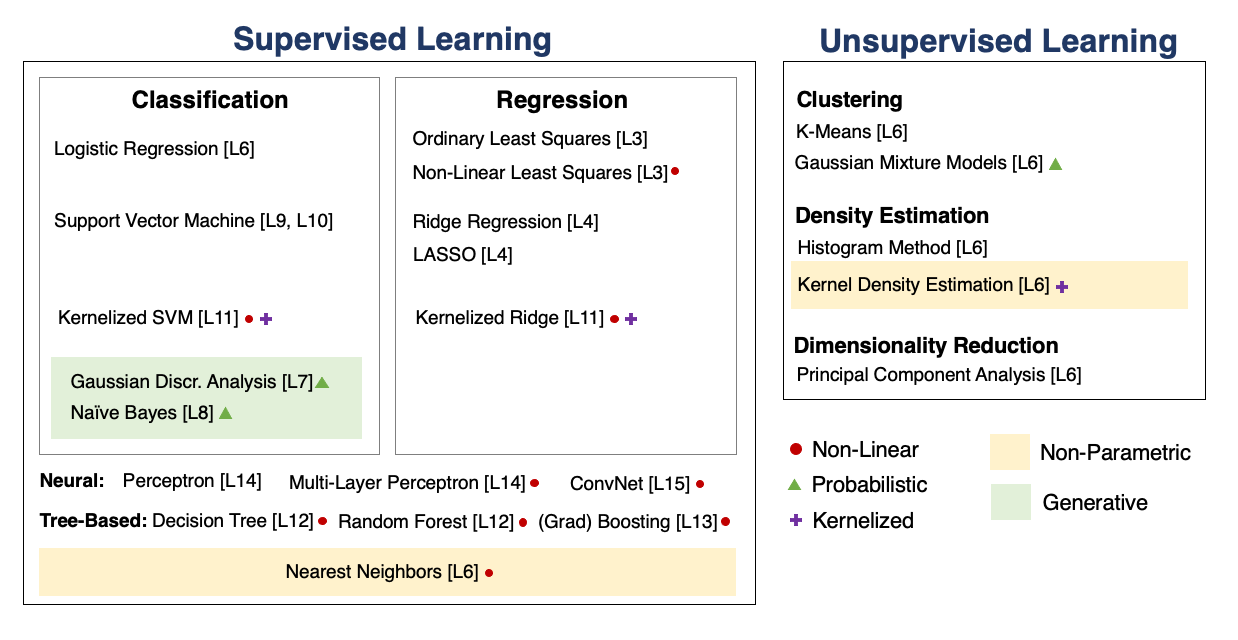# Supervised Machine Learning¶

At a high level, a supervised machine learning problem has the following structure:

$$\underbrace{\text{Dataset}}_\text{Features, Attributes} + \underbrace{\text{Learning Algorithm}}_\text{Model Class + Objective + Optimizer} \to \text{Predictive Model}$$

The predictive model is chosen to model the relationship between inputs and targets. For instance, it can predict future targets.

# Linear Regression¶

In linear regression, we fit a model $$f_\theta(x) := \theta^\top \phi(x)$$ that is linear in $\theta$.

The features $\phi(x) : \mathbb{R} \to \mathbb{R}^p$ are non-linear may non-linear in $x$ (e.g., polynomial features), allowing us to fit complex functions.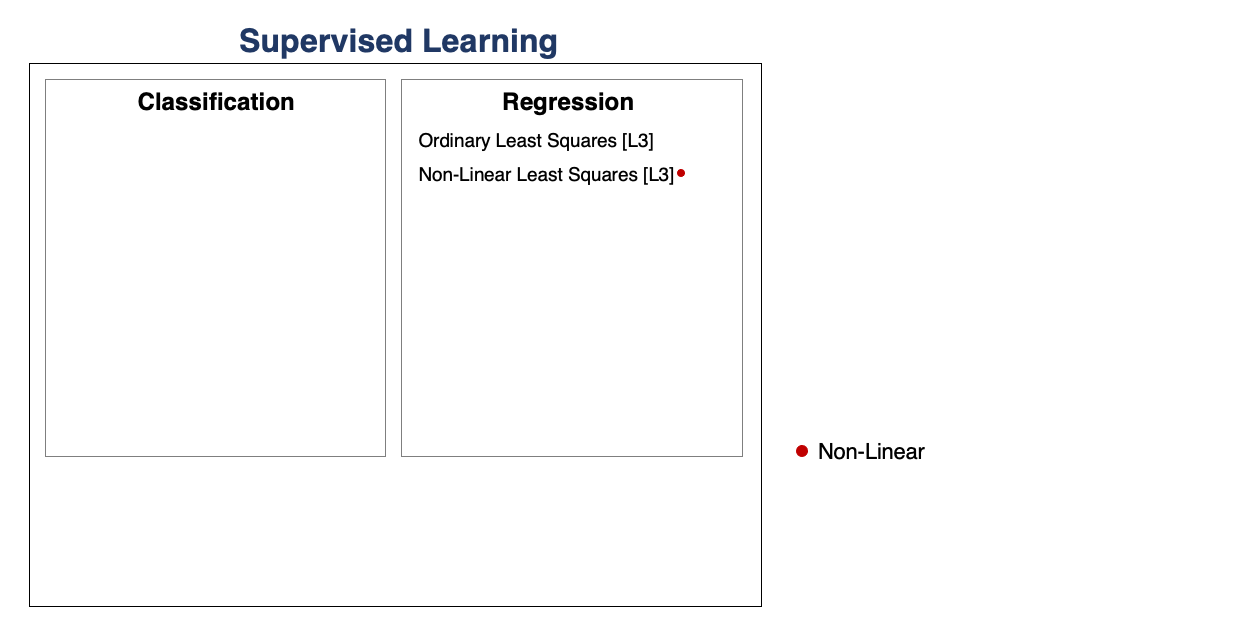# Overfitting¶

Overfitting is one of the most common failure modes of machine learning.

• A very expressive model (a high degree polynomial) fits the training dataset perfectly.
• The model also makes wildly incorrect prediction outside this dataset, and doesn't generalize.

# Regularization¶

The idea of regularization is to penalize complex models that may overfit the data.

Regularized least squares optimizes the following objective (Ridge). $$J(\theta) = \frac{1}{2n} \sum_{i=1}^n \left( y^{(i)} - \theta^\top \phi(x^{(i)}) \right)^2 + \frac{\lambda}{2} \cdot ||\theta||_2^2.$$ If we use the L1 norm, we have the LASSO.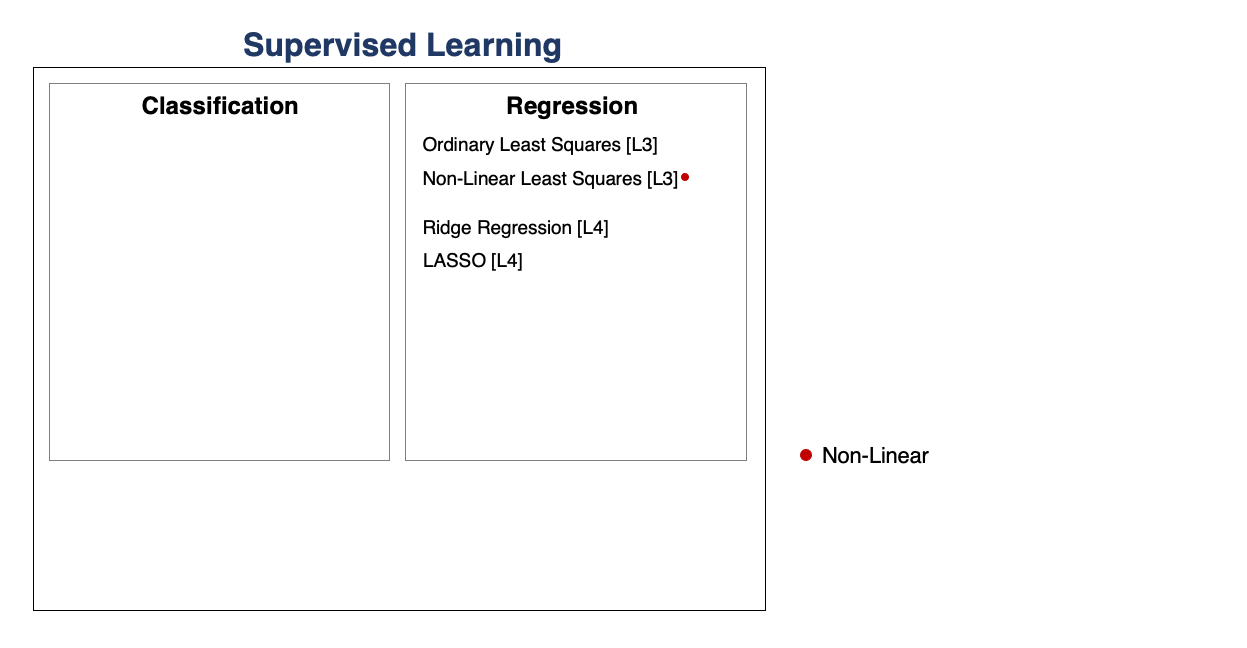# Regression vs. Classification¶

Consider a training dataset $\mathcal{D} = \{(x^{(1)}, y^{(1)}), (x^{(2)}, y^{(2)}), \ldots, (x^{(n)}, y^{(n)})\}$.

We distinguish between two types of supervised learning problems depnding on the targets $y^{(i)}$.

1. Regression: The target variable $y \in \mathcal{Y}$ is continuous: $\mathcal{Y} \subseteq \mathbb{R}$.
2. Classification: The target variable $y$ is discrete and takes on one of $K$ possible values: $\mathcal{Y} = \{y_1, y_2, \ldots y_K\}$. Each discrete value corresponds to a class that we want to predict.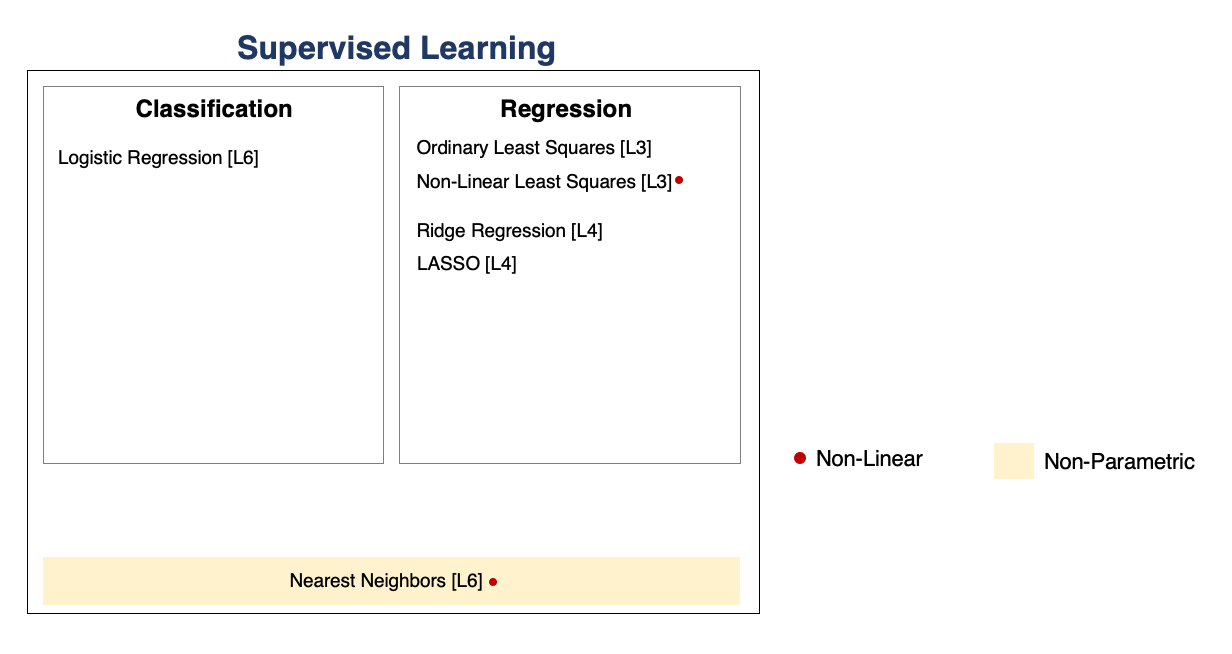# Parametric vs. Non-Parametric Models¶

Nearest neighbors is an example of a non-parametric model.

• A parametric model $f_\theta(x) : \mathcal{X} \times \Theta \to \mathcal{Y}$ is defined by a finite set of parameters $\theta \in \Theta$ whose dimensionality is constant with respect to the dataset
• In a non-parametric model, the function $f$ uses the entire training dataset to make predictions, and the complexity of the model increases with dataset size.
• Non-parametric models have the advantage of not loosing any information at training time.
• However, they are also computationally less tractable and may easily overfit the training set.

# Probabilistic vs. Non-Probabilistic Models¶

A probabilistic model is a probability distribution $$P(x,y) : \mathcal{X} \times \mathcal{Y} \to [0,1].$$ This model can approximate the data distribution $P_\text{data}(x,y)$.

If we know $P(x,y)$, we can use the conditional $P(y|x)$ for prediction.

# Maximum Likelihood Learning¶

Maximum likelihood is an objective that can be used to fit any probabilistic model: $$\theta_\text{MLE} = \arg\max_\theta \mathbb{E}_{x, y \sim \mathbb{P}_\text{data}} \log P(x, y; \theta).$$ It minimizes the KL divergence between the model and data distributions: $$\theta_\text{MLE} = \arg\min_\theta \text{KL}(P_\text{data} \mid\mid P_\theta).$$

# Discriminative vs. Generative Models¶

There are two types of probabilistic models: generative and discriminative. \begin{align*} \underbrace{P_\theta(x,y) : \mathcal{X} \times \mathcal{Y} \to [0,1]}_\text{generative model} & \;\; & \underbrace{P_\theta(y|x) : \mathcal{X} \times \mathcal{Y} \to [0,1]}_\text{discriminative model} \end{align*}

We can obtain predictions from generative models via $\max_y P_\theta(x,y)$.